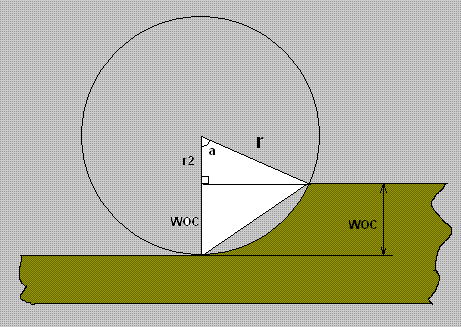Resources and Software For High-Performance CNC Machinists

Categories
Subscribe to Blog

Here i will show you how to calculate Tool Engagement Angle using tool diameter and Width Of Cut (radial deopth of cut)

Lets first draw a pretty image that shows us everything we need.Where:

• r: Radius of the cutter = Diamater /2
• a: TEA - Enagagement angle we are trying to find here
• WOC: Width of cut or RADIAL Depth of Cut
• r2: The difference between r and WOC, r=r2+WOC

Below we develop 2 formulas that allow us to find TEA and WOC

### Task 1: Find WOC (Radial Depth of Cut) knowing tool engagement angle and diameter of the tool

Solution:

WOC=r-r2

r2=COS(a) * r

COS(a)=r2 / r

r2=COS(a) * R

 Diamater Diameter WOC = - COS(a) * 2 2

or if we move Diameter/2 outside of brackets:

 Diamater | | WOC = * | 1- COS(a) | 2 | |

or if we replace Diameter/2 with radius:

WOC=r * (1-COS(a))

### Task 2: Find engagement angle knowing WOC (Radial Depth of Cut) and diameter of the tool

Solution:

WOC =  r - r2

r2 = COS(a) * r

COS(a)= r2 / r

a = COS-1( r2 / r )

a = COS-1( ( r - WOC) / r )

a = COS-1( 1 - WOC / r )

or

a = COS-1( 1 - WOC / Dia/2 )

Thats it folks

You shall be surprised but those two formulas work also for TEA bigger than 90 Degree

Calculating Tool Engagement Angle, Radial Depth of Cut

Pages:(1)#### Divyang

April 9, 2015, 10:30 pm

Nice, Thanks. Could you please show how to Â calculate cutter engagement angle when a tool is performing pocket machining using a curvilinear or spiral like tool path.#### Eldar Gerfanov

April 10, 2015, 11:39 pm
Updated by: Eldar GerfanovApril 10, 2015, 11:43 pm

Quote:
Nice, Thanks. Could you please show how to Â calculate cutter engagement angle when a tool is performing pocket machining using a curvilinear or spiral like tool path.
Â
Â hi,
That is a hard task because your effective with of cut will change with the radius of the toolapth.
It is easy to calculate, but the radius of the toolpath will be constanlty increasing, so your calculations will be off.
Basically the same formulae will apply, but you have to multiply by factor
Fx=(D_Toolpath-D_Cutter)/D_Toolpath
Since your D_Toolpath will be constantly increasing, effect of the spiral radius will be anywhere from about 0.1 to 1#### Divyang

May 2, 2015, 4:46 am

Hi,Â
Thank you very much Eldar Gerfanov for the reply. But still it is very difficult for me to comprehend what you exactly want to say.Â
My question is very simple. Say, I want to machine a circular pocket (with diameter, D) and tool (diameter, d) with spiral toolpath toolpath (R,theta), where R is the radius for corresponding theta. For one convolution theta varies from 0 to 230 degrees. and step over is kept as say 'S'. Â How to calculate the cutter engagement angle in this case. I have tried but things are getting complicated for me as for each pass the surface generated by the preceding convolution has to be taken into account. If possible, a rough sketch would be helpful.Â
once again thanks.#### Eldar Gerfanov

May 3, 2015, 2:03 pm
Updated by: Eldar GerfanovMay 3, 2015, 2:06 pm

Quote:
Hi,Â
Thank you very much Eldar Gerfanov for the reply. But still it is very difficult for me to comprehend what you exactly want to say.Â
My question is very simple. Say, I want to machine a circular pocket (with diameter, D) and tool (diameter, d) with spiral toolpath toolpath (R,theta), where R is the radius for corresponding theta. For one convolution theta varies from 0 to 230 degrees. and step over is kept as say 'S'. Â How to calculate the cutter engagement angle in this case. I have tried but things are getting complicated for me as for each pass the surface generated by the preceding convolution has to be taken into account. If possible, a rough sketch would be helpful.Â
once again thanks.
Â Â
Â That is exactly what i tried to tell you in my previous post.#### Jim

September 15, 2015, 10:41 pm

I'm sure this is not where I'm supposed to post, but I hope somone can help me.Â
I'm having problems opening/installing HSM Advisor in 64 bit vista.Â
Comapatibility mode is un usefull. Any help is much appreciated.#### Eldar Gerfanov

September 16, 2015, 5:12 am

Quote:
I'm sure this is not where I'm supposed to post, but I hope somone can help me.Â
I'm having problems opening/installing HSM Advisor in 64 bit vista.Â
Comapatibility mode is un usefull. Any help is much appreciated.

Â what kind of trouble?
Does it install and then don't run?

# New Comment to: Calculating Tool Engagement Angle, Radial Depth of Cut

Name: *
Security Code:*

Sing In